Nonequispaced fast Fourier transform
NFFT on the sphere
NFSFT

# NFSFT - NFFT on the sphere

This library of C functions computes evaluates a function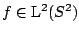with finite orthogonal expansion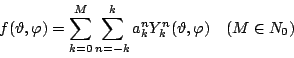in terms of spherical harmonics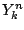on a set of arbitary nodes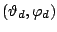,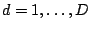,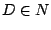, in spherical coordinates. Furthermore, the fast evaluation of sums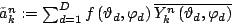for given function values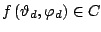and all indices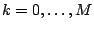,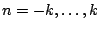is possible. The algorithm is also kwnon as fast spherical harmonic transform for nonequispaced data.

The algorithms are implemented by Jens Keiner in ./kernel/nfsft. Related paper areGräf, M., Kunis, S., Potts, D.
On the computation of nonnegative quadrature weights on the sphere.
Appl. Comput. Harm. Anal., accepted,   (full paper ps, pdf),   2009Keiner, J., Potts, D.
Fast evaluation of quadrature formulae on the sphere
Math. Comput. 77, 397 - 419,   (full paper ps, pdf),   2008Kunis, S. and Potts, D.
Fast spherical Fourier algorithms.
J. Comput. Appl. Math. 161, 75-98. (full paper ps, pdf),   2003Potts, D., Steidl G., and Tasche M.
Fast and stable algorithms for discrete spherical Fourier transforms.
Linear Algebra Appl. 275, 433-450. (full paper ps.Z, pdf),   1998